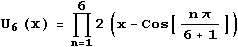This header plots the critical line of the Riemann Zeta Function.  A complete understanding wins a \$1,000,000 prize.
 . . .Main Links Orders Post Next Page Next + 10

Chebychev Polynomials were used by Bill Daly and Steven Stadnicki to solve a problem.   I've built a TRIANGLE page for the results, with new contributions by Bob Harris, Roger Phillips, and many others.

The MacTutor History of Mathematics archive gives Pafnuty Lvovich Chebyshev's biography.  At mathworld.wolfram.com, there are twenty different entries for Chebyshev, including Chebyshev Polynomial of the First Kind and Chebyshev Polynomial of the Second Kind.  What do they mean?  I gained my first insight when I plugged cos(Pi/9) into the Inverse Symbolic Calculator.  The Sixth Chebyshev Polynomial of the Second Kind is -1 + 24 x2 - 80 x4 + 64 x6. In Mathematica, ChebyshevU[6,x].  This polynomial is also expressed as U6(x).

ChebyshevU[6,x] = -1 + 24 x2 - 80 x4 + 64 x6 = - (1 + 4 x - 4 x2 - 8 x3) (1 - 4 x - 4 x2 + 8 x3)
-(1 + 4 x - 4 x2 - 8 x3) / 8  = (x - cos(2p/7)) (x - cos(4p/7)) (x - cos(6p/7))
(1 - 4 x - 4 x2 + 8 x3) / 8  = (x - cos(p/7)) (x - cos(3p/7)) (x - cos(5p/7))Thus, this polynomial is closely related to the heptagon.  Similarly, 1 - 6 x + 8 x3 is closely related to the nonagon, as a factor of U8(x).  In general, the following is true ... but I don't see at Mathworld, or in my references.Please visit the Mathworld link (Chebyshev Polynomial of the Second Kind) to see more representations.  A useful recurrence relationship: U0(x) = 1. U1(x) = 2xUk+2(x) = 2 x Uk+1(x) - Uk(x).  This is easily proved by induction if you use the matrix representation, but would likely be tricky using the product of cosines relationship.